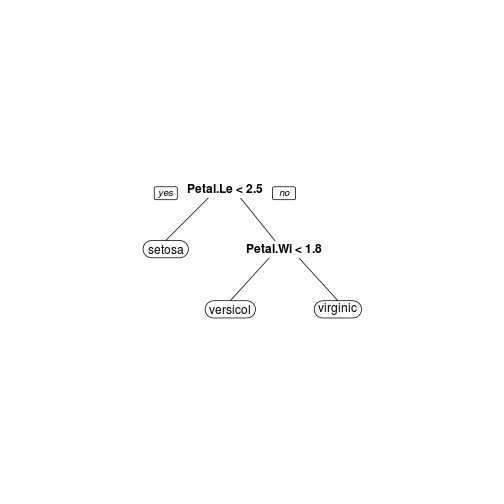Want to share your content on R-bloggers? click here if you have a blog, or here if you don't.

What are trees?

Trees (also called decision trees, recursive partitioning) are a simple yet powerful tool in predictive statistics. The idea is to split the covariable space into many partitions and to fit a constant model of the response variable in each partition. In case of regression, the mean of the response variable in one node would be assigned to this node. The structure is similar to a real tree (from the bottom up): there is a root, where the first split happens. After each split, two new nodes are created (assuming we only make binary splits). Each node only contains a subset of the observations. The partitions of the data, which are not split any more, are called terminal nodes or leafs. This simple mechanism makes the interpretation of the model pretty easy.

Interpretation looks like: “If $$x1 > 4$$ and $$x2 < 0.5$$ than $$y = 12$$." This is much easier to explain to  a non-statistician than a linear model. Therefore it is a powerful tool not only for prediction, but also to explain the relation of your response $$Y$$ and your covariables $$X$$ in an easy understandable way.

Different algorithms implement these kind of trees. They differ in the criterion, which decides how to split a variable, the number of splits per step and other details. Another difference is how pruning takes places. Pruning means to shorten the tree, which makes trees more compact and avoids overfitting to the training data. The algorithms have in common, that they all use some criterion to decide about the next split. In case of regression, the split criterion is the sum of squares in each partition. The split is made at the variable and split point, where the best split can be achieved according to the criterion (regression trees: minimal sum of squares)

To avoid too large trees, there are two possible methods:

1. Avoid growing large trees: This is also called early stopping. Stopping criteria might be, that the number of observations in a node undercuts some minimum number of observations. If the criterion is fulfilled the current node will not be split any further. Early stopping yields smaller trees and saves computational time.

2. Grow large tree, cut afterwards: Also known as pruning. The full tree is grown (early stopping might additionally be used), and each split is examined, if it brings a reliable improvement. This can be top-down, starting from the first split made, or bottom-up, starting at the splits above the terminal nodes. Bottom-up is more common, because top-down has the problem, that whole sub-trees can be trashed. However, after a "bad” split a lot of good splits can follow. Pruning takes into account the weighted split criterion for all splits and the complexity of the trees, which is weighted by some $$\alpha$$. Normally the complexity parameter $$\alpha$$ is chosen data-driven by cross-validation.

How can I use those trees?

The R package rpart implements recursive partitioning. It is very easy to use. The following example uses the iris data set. I'm trying to find a tree, which can tell me if an Iris flower species is setosa, versicolor or virginica, using some measurements as covariables. As the response variable is categorial,  the resulting tree is called classification tree. The default criterion, which is maximized in each split is the gini coefficient. The model, which is fit to each node, is simply the mode of the flower species, the flower which appears most often in this node.

The result is a very short tree: If Petal.length is smaller than 2.4 we label the flower with setosa. Else we look at the covariable Petal.Width. Is Petal.Width smaller than 1.8? If so, we label the flower versicolor, else virginica.

I personally think the plots from the rpart package are very ugly, so I use the plot function rpart.plot from the rpart.plot package. The results from the tree show, that all of the Iris flowers which are in the left node are correctly labeled setosa, no other flower is in this terminal node of the tree. The other terminal nodes are also very pure, the versicolor labeled node contains 54 correctly assigned flowers and 5 wrongly assigned. The virginic node has about the same purity (46 correctly, 1 incorrectly assigned).

library("rpart")library("rpart.plot")data("iris")tree <- rpart(Species ~ ., data = iris, method = "class")rpart.plot(tree)tree
## n= 150 ## ## node), split, n, loss, yval, (yprob)##       * denotes terminal node## ## 1) root 150 100 setosa (0.33333 0.33333 0.33333)  ##   2) Petal.Length< 2.45 50   0 setosa (1.00000 0.00000 0.00000) *##   3) Petal.Length>=2.45 100  50 versicolor (0.00000 0.50000 0.50000)  ##     6) Petal.Width< 1.75 54   5 versicolor (0.00000 0.90741 0.09259) *##     7) Petal.Width>=1.75 46   1 virginica (0.00000 0.02174 0.97826) *

The method-argument can be switched according to the type of the response variable. It is “class”“ for categorial, "anova”“ for numerical, "poisson”“ for count data and "exp”“ for survival data.
All in all the package is pretty easy to use. Thanks to the formula interface, it can be used like most other regression models (like lm(), glm() and so on). I personally think the utility of trees as regression models is underestimated. They are super-easy to understand and if you have to work with non-statistician it might be a benefit to use trees.